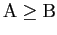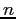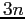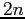# Sequential Circuit Design

Tom Kelliher, CS 240

Apr. 9, 2012

### From Last Time

Sequential circuit analysis.

### Outline

1. Sequential circuit design process.

2. Unused states.

3. Examples.

### Coming Up

VHDL for sequential circuits.

# Sequential Circuit Design Process

1. Obtain a state diagram. Assign binary numbers to the states (a non-trivial problem, actually).

2. Obtain a state table.

3. Derive flip-flop input equations from the next state entries and output equations. Simplify.

# Unused States

Suppose your design has 6 states:

1. Two unused states.

2. What happens if the circuit enters one of these states?

# Examples

1. Sequence recognizer for 010.

2. Serial comparator. Inputs: A, B, msb. A and B are received least significant bit first. Receipt of msb is co-incident with msb's of A and B and resets circuit to begin next comparison. Output 0 if, otherwise 1.

3. Serial comparator. Inputs: A, B, lsb. A and B are received most significant bit first. Receipt of lsb is co-incident with lsb's of A and B and resets circuit to begin next comparison. Output 0 if, otherwise 1.

4. Given an unsigned binary valueserially, starting from the lsb, compute. Hint: How would you compute?

Thomas P. Kelliher 2012-04-07
Tom Kelliher